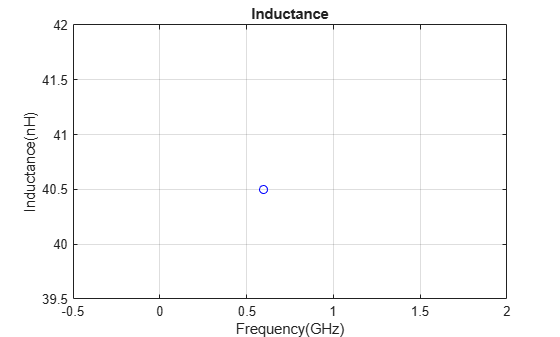# inductance

Calculate inductance

Since R2021b

## Syntax

``inductance(object,frequency)``
``l = inductance(object,frequency)``

## Description

example

````inductance(object,frequency)` calculates and plots the inductance of an inductor over the specified frequency.```
````l = inductance(object,frequency)` calculates the inductance of an inductor over the specified frequency.```

## Examples

collapse all

Create a hexagonal spiral inductor with default properties.

```inductor = spiralInductor(SpiralShape='Hexagon'); show(inductor)```Calculate the inductance of the inductor at 600 MHz.

`inductance(inductor,600e6)``l = inductance(inductor,600e6)`
```l = 3.8052e-08 ```

## Input Arguments

collapse all

Spiral inductor, specified as an `spiralInductor` object.

Data Types: `char` | `string`

Frequency to calculate inductance, specified as a nonnegative scalar or vector in hertz.

Data Types: `double`

## Output Arguments

collapse all

Inductance of the inductor, returned as a scalar or vector in henries.

Data Types: `double`

## Version History

Introduced in R2021b